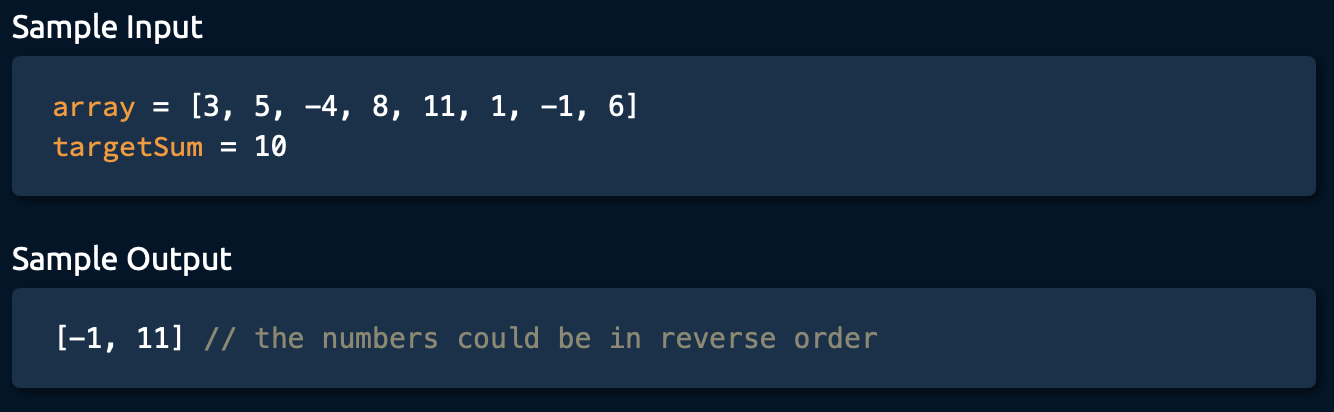# Array Algorithm 11435-郭同学

, , ,

# Two Number Sum

Write a function that takes in a non-empty array of distinct integers and an integer representing a target sum. If any two numbers in the input array sum up to the target sum, the function should return them in an array, in any order. If no two numbers sum up to the target sum, the function should return an empty array.
Note that the target sum has to be obtained by summing two di!erent integers in the array; you can’t add a single integer to itself in order to obtain the target sum.
You can assume that there will be at most one pair of numbers summing up to the target sum.Solution 1:
time complexity : O(n^2)

def twoNumberSum(array, targetSum):
solution = []
for i in range(len(array) - 1):
currentNum = array[i]
for j in range(i + 1,len(array)):
secondNum = array[j]
if currentNum + secondNum == targetSum:
if currentNum > secondNum:
return [secondNum, currentNum]
else:
return [currentNum, secondNum]
return []


Solution 2:
Hashing
time complexity : O(n)

def twoNumberSum(array, targetSum):
nums = {}
for num in array:
if targetSum - num in nums:
return [targetSum - num, num]
else:
nums[num] = True
return []


Solution 3:
Sort
time complexity : O(nlogn)

def twoNumberSum(array, targetSum):
array.sort()
left = 0
right = len(array) - 1
while left < right:
currentSum = array[left] + array[right]
if currentSum == targetSum:
return [array[left], array[right]]
elif currentSum > targetSum:
right -= 1
else:
left += 1
return []


from AlgoExpert

### 评论 抢沙发### 觉得文章有用就打赏一下文章作者

#### 支付宝扫一扫打赏#### 微信扫一扫打赏Vieu3.3主题Q Q 登 录